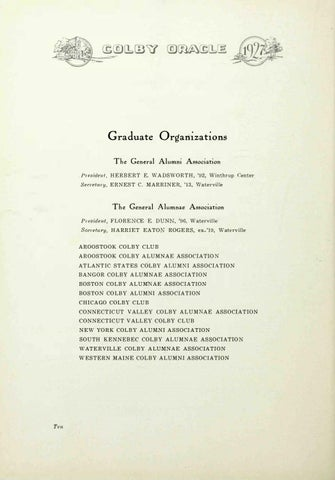Graduate Organizations The General Alumni Association President,

H E R B E R T E. WA D S WO R TH , ' 9 2 , Wi nthrop

Secretmy,

E R N E S T C. M A R R I N E R, ' 1 3 ,

v

enter

aterville

The General Alumnae Association President,

FLORE

CE E. D U N

S creta1Â·y, H A R R I E T E A TO

, '96, Watervi l l e

R O G E R S , ex- ' 1 9 , Waterv i l l e

A R O O S TO O K CO L B Y CLU B A R O O S TO O K CO L B Y A L U M N A E A S S O C I A TI O A T L A N T I C S TA TE S C O L B Y A LU M NI A S S O C I ATI O N B A N G O R CO L B Y A L U M N A E A S S O CI A TI O N B O S TO N CO L B Y A L U MNA E A S S O CI A TI O N B O S TO N CO L B Y A L U M N I A S SO C IA TI O N C H I CA GO C O L B Y CLU B C O N N E CTICUT V A L L E Y CO L B Y A L U M

A E A S S O CI A TI O N

C O N N E CTI CU T V A L L E Y C O L B Y C L U B N E W YO R K CO L B Y A L U M N I A S S O CI A TI O N S O U TH K E N N E B E C CO L B Y A L U M N A E A S SO C I A TI O WATE R V I L L E CO L B Y A LU M N A E A S S O C IA TI O N W E S TE R N M A I N E CO L B Y A L U M N I A S S O C I A TI O N

Ten